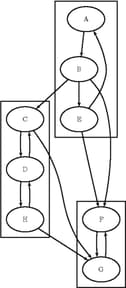### Connected Components

A connected component of a graph is a set of nodes that are all reachable from each other. That is, if two nodes are in the same component, then there exists a path between them. For a directed graph, there are two types of components: a strongly connected component has a directed path between any two nodes, and a weakly connected component ignores direction and requires only that a path exists between any two nodes.

In PROC OPTNET, connected components can be invoked by using the CONCOMP statement. The options for this statement are described in the section CONCOMP Statement.

There are two main algorithms for finding connected components on an undirected graph: a depth-first search algorithm (ALGORITHM=DFS) and a union-find algorithm (ALGORITHM=UNION_FIND). Given a graph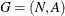, both algorithms run in time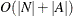and typically can scale to very large graphs. The default, depth-first search, works only with a full graph structure (GRAPH_INTERNAL_FORMAT=FULL) and for this reason can sometimes be slower than the union-find algorithm. For directed graphs, the only algorithm available is depth-first search.

The results for the connected components algorithm are written to the output node data set that is specified in the OUT_NODES= option in the PROC OPTNET statement. For each node in the node data set, the variable `concomp` identifies its component. The component identifiers are numbered sequentially starting from 1.

#### Connected Components of a Simple Undirected Graph

This section illustrates the use of the connected components algorithm on the simple undirected graph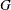shown in Figure 2.20.

Figure 2.20: A Simple Undirected Graph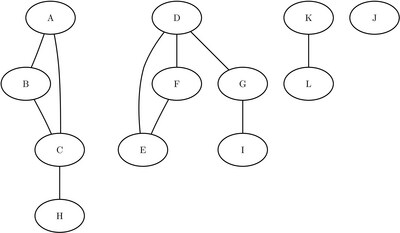The undirected graphcan be represented by the links data set `LinkSetIn` as follows:

```data LinkSetIn;
input from \$ to \$ @@;
datalines;
A B  A C  B C  C H  D E  D F  D G  F E  G I  K L
;
```

The following statements calculate the connected components and output the results in the data set `NodeSetOut`:

```proc optnet
out_nodes  = NodeSetOut;
concomp;
run;
```

The data set `NodeSetOut` contains the connected components of the input graph and is shown in Figure 2.21.

Figure 2.21: Connected Components of a Simple Undirected Graph

node concomp
A 1
B 1
C 1
H 1
D 2
E 2
F 2
G 2
I 2
K 3
L 3

Notice that the graph was defined by using only the links data set. As seen in Figure 2.20, this graph also contains a singleton node labeled J, which has no associated links. By definition, this node defines its own component. But because the input graph was defined with the links data set alone, it did not show up in the results data set. To define a graph with nodes that have no associated links, you should also define the input nodes data set. In this case, define the nodes data set `NodeSetIn` as follows:

```data NodeSetIn;
input node \$ @@;
datalines;
A  B  C  D  E  F  G  H  I  J  K  L
;
```

Now, when you calculate the connected components, you define the input graph by using both the nodes and links input data sets:

```proc optnet
data_nodes = NodeSetIn
out_nodes  = NodeSetOut;
concomp;
run;
```

The resulting data set `NodeSetOut` includes the singleton node J as its own component, as shown in Figure 2.22.

Figure 2.22: Connected Components of a Simple Undirected Graph

node concomp
A 1
B 1
C 1
D 2
E 2
F 2
G 2
H 1
I 2
J 3
K 4
L 4

#### Connected Components of a Simple Directed Graph

This section illustrates the use of the connected components algorithm on the simple directed graphshown in Figure 2.23.

Figure 2.23: A Simple Directed Graph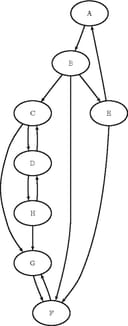The directed graphcan be represented by the links data set `LinkSetIn` as follows:

```data LinkSetIn;
input from \$ to \$ @@;
datalines;
A B  B C  B E  B F  C G
C D  D C  D H  E A  E F
F G  G F  H G  H D
;
```

The following statements calculate the connected components and output the results in the data set `NodeSetOut`:

```proc optnet
graph_direction = directed
out_nodes       = NodeSetOut;
concomp;
run;
```

The data set `NodeSetOut`, shown in Figure 2.24, now contains the connected components of the input graph.

Figure 2.24: Connected Components of a Simple Directed Graph

node concomp
A 3
B 3
C 2
E 3
F 1
G 1
D 2
H 2

The connected components are represented graphically in Figure 2.25.

Figure 2.25: Strongly Connected Components of